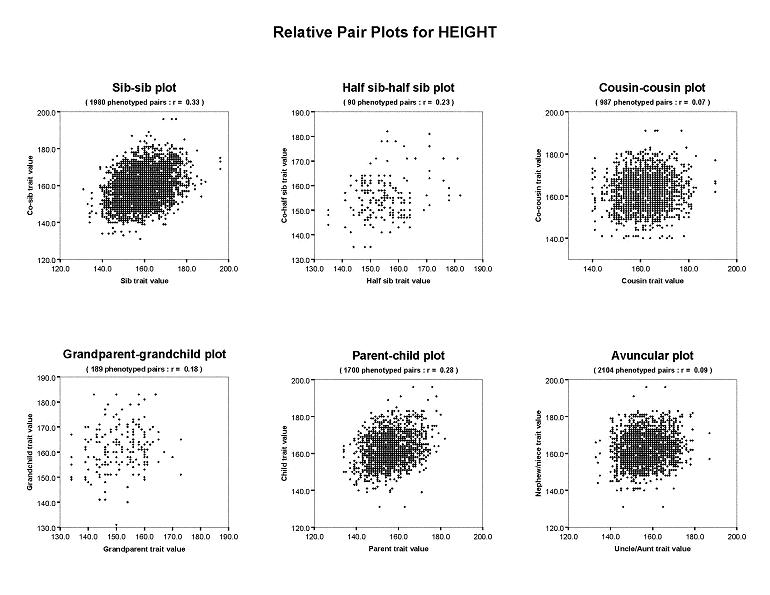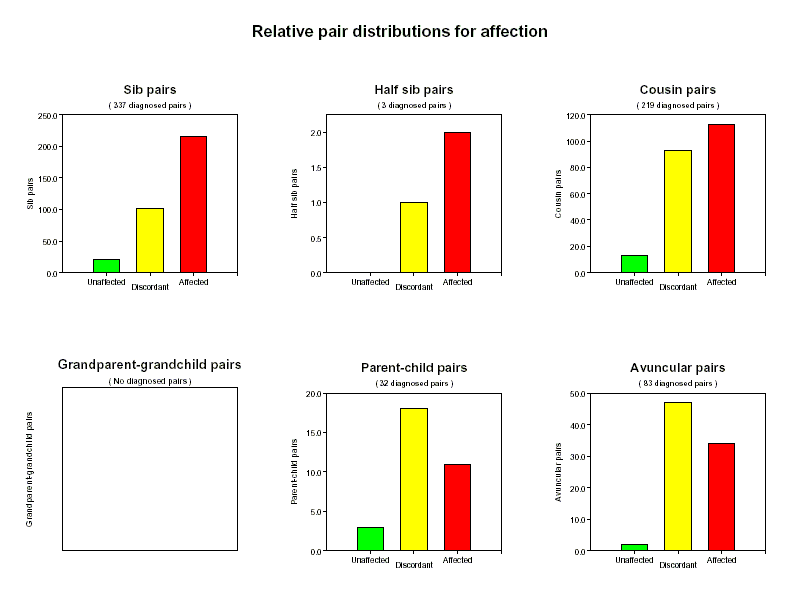## PEDSTATS Tutorial -- Pairwise Distributions

When the --pairStatistics option is used in conjunction with the --pdf option, PEDSTATS appends its standard pdf output with a page of charts summarizing pairwise distributions among first and second degree relative pairs for each quantitative trait, covariate, or affection variable in your pedigree file.

### Pairwise Summary of Covariate and Quantitative Traits

For quantitative traits and covariates, the ploats are arranged in two rows, each with three plots. In Figure 1, the upper row contains scatter plots showing the pairwise distribution of HEIGHT for unordered pair types (sib, half-sib and first cousin pairs), while the bottom row contains the same information for each pair relationship that has an inherent ordering (grandparent-grandchild, parent-child and avuncular relationships). Pair types in the top row are plotted symmetrically with 2 data points per pair. Values for ordered pair types are represented by the single data point formed by plotting the ancestor value along the x-axis and the descendant value along the y-axis. For all pair types, the number of phenotyped pairs and the correlation are given in the plot title.### Pairwise Summary of Dichotomous Variables

For dichotomous traits, a breakdown of affection status similar to that shown in Figure 2 is given for each relative pair type. Here, each chart shows the number of affected, unaffected and discordant pairs for the corresponding relative pair type.University of Michigan | School of Public Health